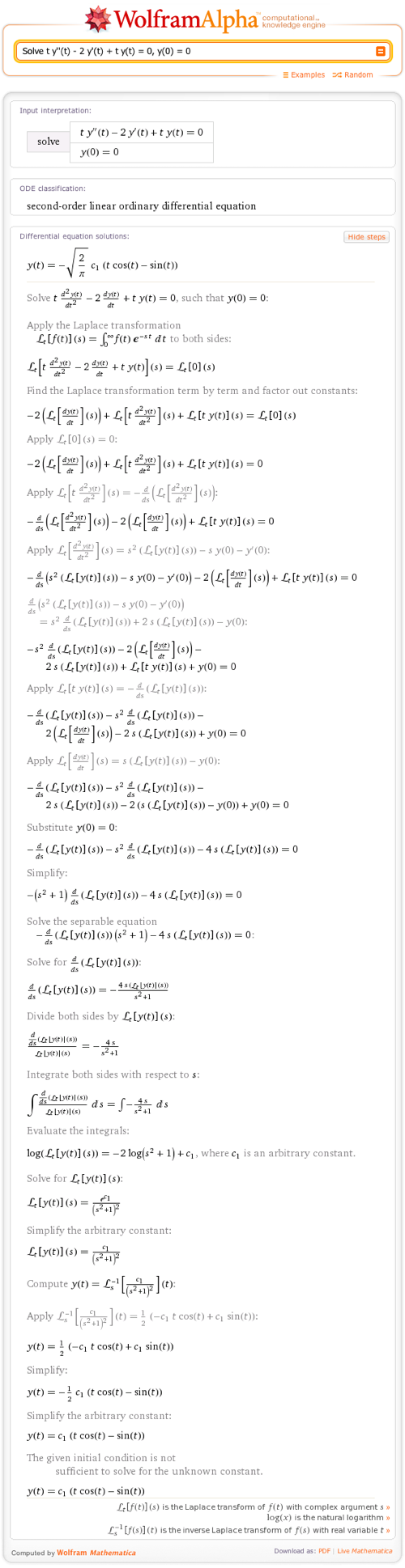# Step-by-Step Differential Equation Solutions in Wolfram|Alpha

January 30, 2012 —Comments Off

Wolfram|Alpha has become well-known for its ability to perform step-by-step math in a variety of areas. Today we’re pleased to introduce a new member to this family: step-by-step differential equations. Differential equations are fundamental to many fields, with applications such as describing spring-mass systems and circuits and modeling control systems.

From basic separable equations to solving with Laplace transforms, Wolfram|Alpha is a great way to guide yourself through a tough differential equation problem. Let’s take a look at some examples.

Wolfram|Alpha can show the steps to solve simple differential equations as well as slightly more complicated ones like this one:

Wolfram|Alpha can help out in many different cases when it comes to differential equations. Get step-by-step directions on solving exact equations or get help on solving higher-order equations. Even differential equations that are solved with initial conditions are easy to compute.

What about equations that can be solved by Laplace transforms? Not a problem for Wolfram|Alpha:This step-by-step program has the ability to solve many types of first-order equations such as separable, linear, Bernoulli, exact, and homogeneous. In addition, it solves higher-order equations with methods like undetermined coefficients, variation of parameters, the method of Laplace transforms, and many more. So the next time you find yourself stuck solving a differential equation or wanting to check your work, consult Wolfram|Alpha!

Nice work Greg!

Posted by Sam Blake January 30, 2012 at 10:11 pm

Hi.

Is this option also available on Mathematica or it’s just for the WolframAlpha?

Posted by José Carlos Méndez January 31, 2012 at 4:15 am

Muy util. En aplicaciones como diseño de filtros en electronica y en diseño de sistemas de control. Muchas gracias.

Posted by JUAN January 31, 2012 at 10:58 am

Bearing in mind that the target is to add everything to Wolfram Alpha with a view to getting as near as practicable…I suggest that the emphasis be put on Wolfram Alpha generating the Step by Step information from the actual steps it takes to arricve at its solution. As it is the work involved will prove a significant limiting factor.

Posted by Brian Gilbert January 31, 2012 at 6:29 pm

I have a problem with the input of the ( ‘ ) to write the Differential equation in the android app. Any solution?

Posted by Oriol February 1, 2012 at 3:57 pm

Well, one workaround is to use the generic Android keyboard, which also has the advantage of Swype support. You can change this under Menu -> More -> Preferences.

Posted by Bhuvanesh February 3, 2012 at 8:56 pm

search the android market for “Hacker’s Keyboard” – it has just about every symbol you can think of.

Posted by rymo February 7, 2012 at 3:40 pm

My teacher was very impressed with this when I showed it to him, little did I know it was such a new feature.
I know that this request is propably way under your league, but what about showing steps when solving two equations with two unknowns, or three EQ with three unknowns etc.. ?

Thanks for a great piece of tech.!

Posted by Peter Helstrup Jensen February 5, 2012 at 8:23 am

whats the procedure for partial differential equation ??

Posted by Owais May 13, 2012 at 7:45 am

is it possible to make wolfram solve a problem using an specific method like D operator method etc??? if yes plz explain how?!

Posted by Cna SamPaD August 14, 2012 at 8:34 am

integrate t.5^t/2

Posted by Guido May 12, 2014 at 5:23 am

Neither the app version of wolfram alpha nor pro supports step by step solutions for laplace transformations, inverse laplace, or convolutions as of June 17th 2014 as claimed

Posted by Bryful June 17, 2014 at 3:05 pm

Hello, thank you for your comment! Are you trying to solve an ODE? If so, it will show partial steps for Laplace and InverseLaplace.

Posted by The Wolfram|Alpha Team June 18, 2014 at 4:49 pm

(y”(x))^3+(1-x)+(y”(x))^2+1=0

Posted by ali September 9, 2014 at 2:19 pm

For the Differential equation, y”-y+x=0
How is the lagrangian determined?

Posted by mahima October 7, 2014 at 4:47 am

Thanks for this. How can we interactivity change the boundary conditions ?

Posted by Mazi November 29, 2014 at 11:34 pm

Hi, am presently doing my master program (Concordia University,Montreal Canada ) in the department of math and stat. Mathematical modelling is one of the course I will be doing this winter season and we will be using WOLFRAM ALPHA for the duration of the course. I need a friend that have good indept of the software and to guilde me about the application. I will be very happy to hear back from you vial emails (olusesan.ogunsanya@yahoo.com)
thanks..

Posted by olusesan ogunsanya January 15, 2015 at 1:28 pm